Next: Exercises Up: Keplerian orbits Previous: Hyperbolic orbits

# Binary star systems

Approximately half of the stars in our galaxy are members of so-called binary star systems. Such systems consist of two stars, of mass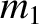and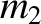, and position vectors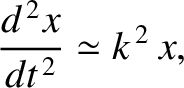and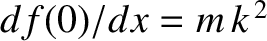, respectively, orbiting about their common center of mass. The distance separating the stars is generally much less than the distance to the nearest neighbor star. Hence, a binary star system can be treated as a two-body dynamical system to a very good approximation.

In a binary star system, the gravitational force that the first star exerts on the second is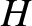(4.108)

where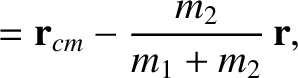. As we have seen in Section 2.9, a two-body system can be reduced to an equivalent one-body system whose equation of motion is of the form given in Equation (2.76), where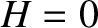. Hence, in this particular case, we can write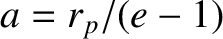(4.109)

which gives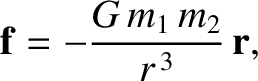(4.110)

where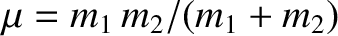(4.111)

Equation (4.110) is identical to Equation (4.2), which we have already solved. Hence, we can immediately write down the solution: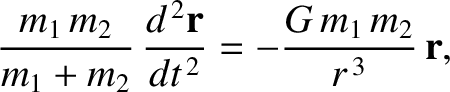(4.112)

where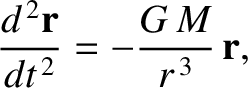(4.113)

and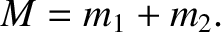(4.114)

with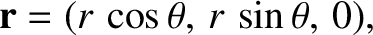(4.115)

Here,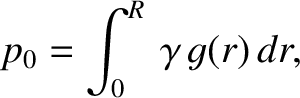is a constant, and we have aligned our Cartesian axes such that the plane of the orbit coincides with the-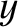plane. According to this solution, the second star executes a Keplerian elliptical orbit, with major radiusand eccentricity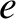, relative to the first star, and vice versa. From Equation (4.33), the period of revolution,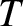, is given by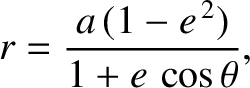(4.116)

Moreover, if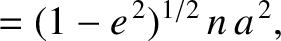then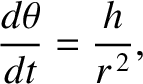(4.117)

In the inertial frame of reference whose origin always coincides with the center of mass--the so-called center of mass frame--the position vectors of the two stars are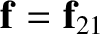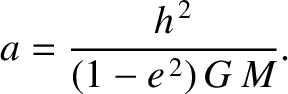(4.118) and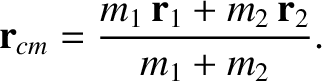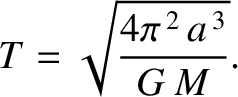(4.119)

where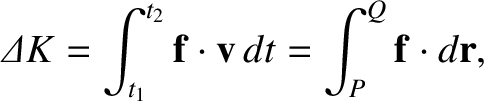is specified in Equation (4.112). Figure 4.8 shows an example binary star orbit, in the center of mass frame, calculated with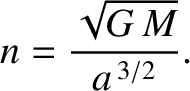and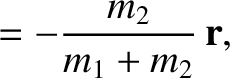. It can be seen that both stars execute elliptical orbits about their common center of mass, and, at any point in time, are diagrammatically opposite one another, relative to the origin.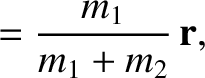Binary star systems are very useful to astronomers, because it is possible to determine the masses of both stars in such a system by careful observation. The sum of the masses of the two stars,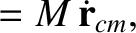, can be found from Equation (4.116) after a measurement of the major radius,(which is the mean of the greatest and smallest distance apart of the two stars during their orbit), and the orbital period,. The ratio of the masses of the two stars,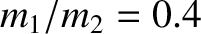, can be determined from Equations (4.118) and (4.119) by observing the fixed ratio of the relative distances of the two stars from the common center of mass about which they both appear to rotate. Obviously, given the sum of the masses, and the ratio of the masses, the individual masses themselves can then be calculated.

Incidentally, an obvious corollary of the material presented in this section is that we can correct the two-body solar-system orbit theory outlined in Sections 4.1-4.15 in order to take into account the finite mass,, of the orbiting body, compared to the solar mass,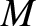, by replacingby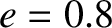wherever it appears in the analysis. For instance, Equation (4.40) generalizes to give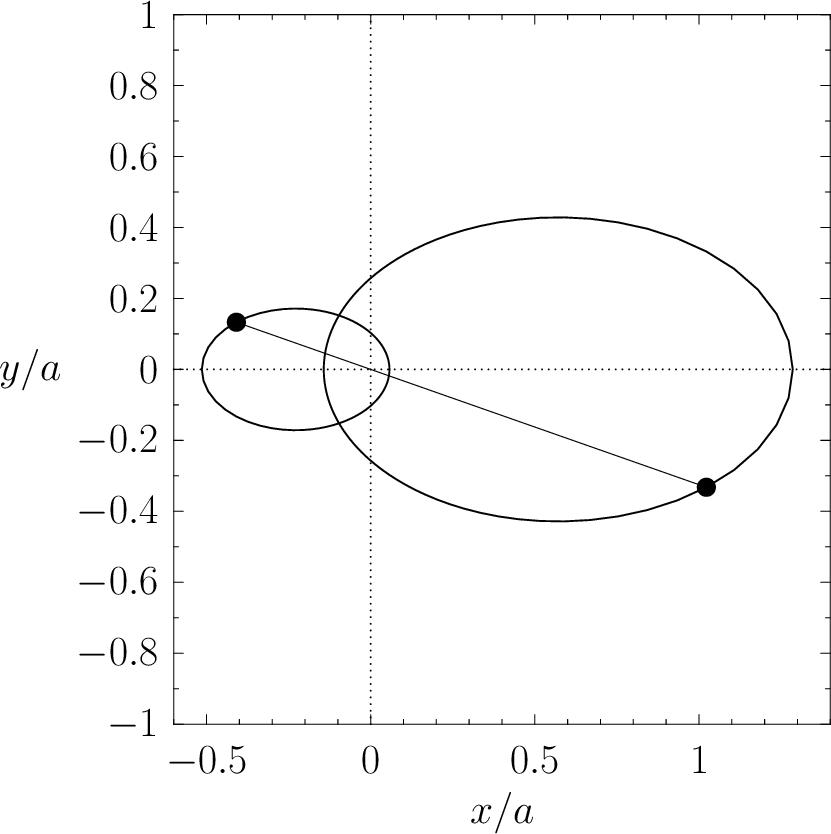.Next: Exercises Up: Keplerian orbits Previous: Hyperbolic orbits
Richard Fitzpatrick 2016-03-31